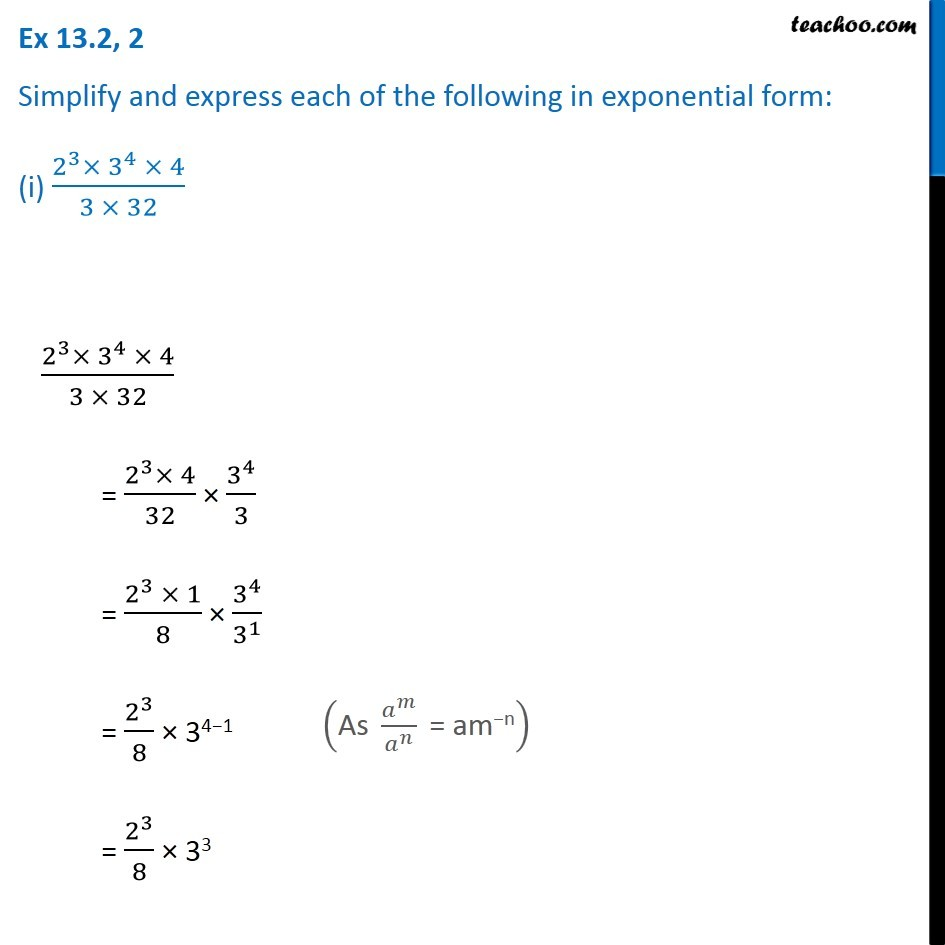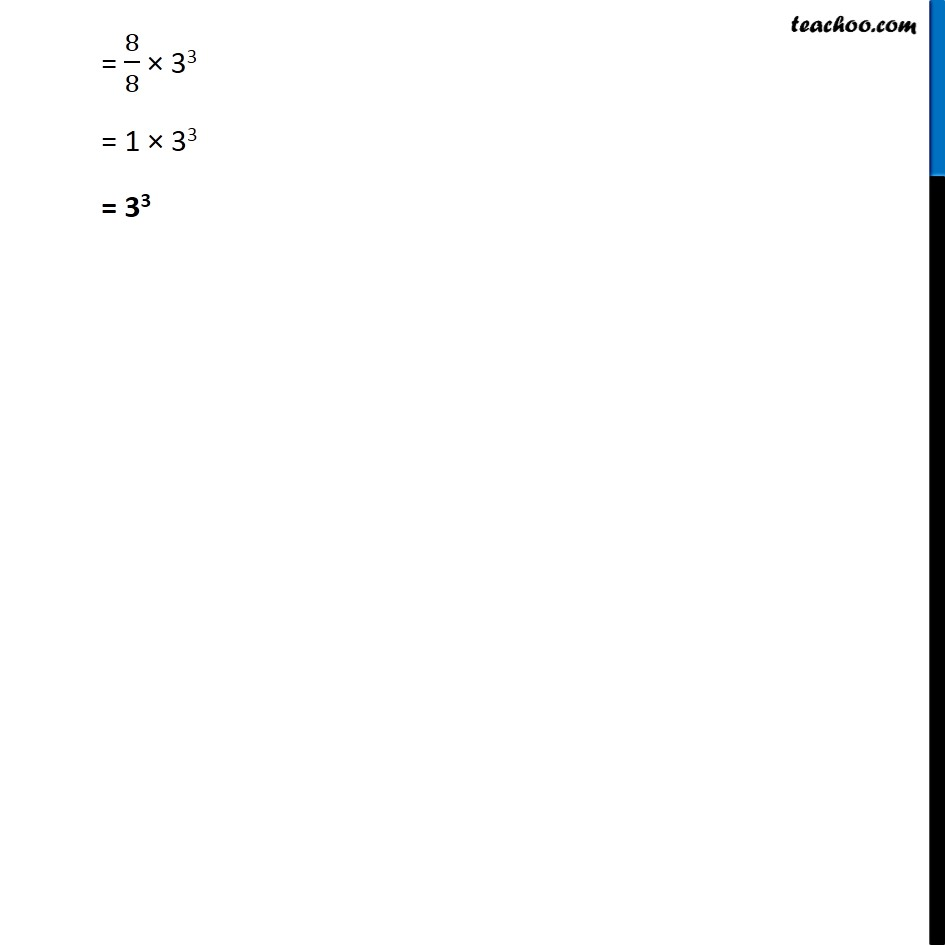1. Chapter 13 Class 7 Exponents and Powers
2. Concept wise
3. Law of exponents

Transcript

Ex 13.2, 2 Simplify and express each of the following in exponential form: (i) (2^3× 3^4 × 4)/(3 × 32) (2^3× 3^4 × 4)/(3 × 32) = (2^3× 4)/32 × 3^4/3 = (2^3 × 1)/8 × 3^4/3^1 = 2^3/8 × 34−1 = 2^3/8 × 33 ("As " 𝑎^𝑚/𝑎^𝑛 " = am−n" ) = 8/8 × 33 = 1 × 33 = 33